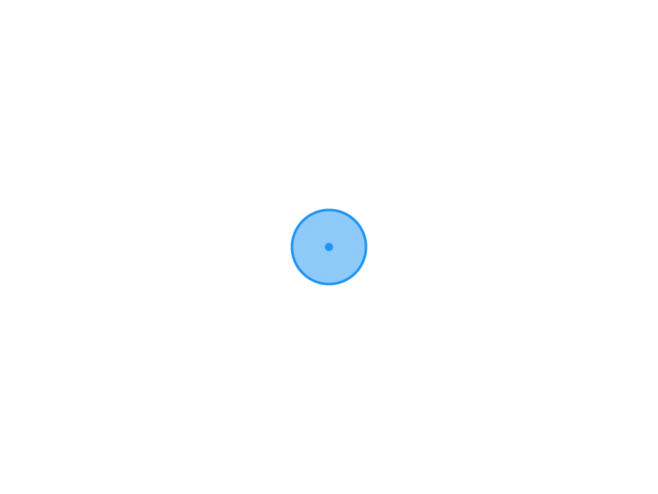TypechoJoeTheme

# 日志随记

/XG.孤梦

# C语言程序的输入与顺序程序设计-学习六2022-01-27
/
0 评论
/
584 阅读
/
740 个字
/

01/27

## 格式输入

• scanf函数
• 一般形式： `scanf(格式控制,地址列表)`

• 格式控制同printf函数的格式控制一致
• 地址列表可以是变量旳地址,或字符串的首地址

• scanf()不会检查输入边界，可能造成数据溢出，scanf_s()会进行边界检查。

• scanf("%s",name),如果输入abcdefgh，则会使得fgh溢出进入其他地方，造成程序错误。
• scanf_s("%s",name),则会只会接收前5个输入，其后的无效，避免了程序的漏洞。

### scanf函数中的格式声明

• 例如： `scanf("a=%f,b=%d,c=%s",&a,&b,&c)`

• “&”是一个取地址运算符&a是一个表达式，其功能是求变量的地址。这个地址是编译系统在内存中给a变量分配的存储空间地址
• 变量的值和变量的地址是两个不同的概念。变量的地址是C编译系统分配的
• 变量的地址和变量值的关系如下:
如：a=123；则a为变量名，123是变量的值,起始地址:1001。#### 例子

``````#include <stdio.h>

void main(){
int a, b, c;
scanf_s("%d%d%d", &a, &b, &c);
printf("%d，%d，%d\n", a, b, c);

}``````#### 使用scanf函数时应注意的问题

1.scanf函数中没有精度控制。

• 错误： `scanf(%7.2f",&a);`
• 正确： `scanf(%f",&a);`

2.scanf涵数中要求给出变量地址，而不是变量名

• 错误： `scanf("%d",a);`
• 正确： `scanf("%d",&a);`

3.若格式控制串中没有非格式字符作输入数据之间的间隔，则可用空格TAB回车作间隔，如果有非格式字符，则在输入时，在对应位置上要输入这些字符。

4.在输入字符数据时，若格式控制串中无非格式字符，则认为所有输入的字符均为有效字符。

5.如果%后有一个“ * ”附加说明符，表示跳过它指定的列数。

``````#include <stdio.h>

void main(){
int a, b, c;
scanf_s("%d,%d,%d", &a, &b, &c);
printf("%d，%d，%d\n", a, b, c);

}````````````#include <stdio.h>
#pragma warning(disable:4996)

void main(){
char a, b, c;
scanf("%c%c%c", &a,&b,&c);
printf("%c，%c，%c\n",a,b,c);

}``````%后有一个“ * ”附加说明符

``````#include <stdio.h>

void main(){
int a, b;
scanf_s("%2d %*3d %d", &a, &b);
printf("%d，%d\n", a, b);

}``````## 顺序结构设计

### 例一

• sqrt函数计算开方
• 使用海伦公式计算三角形的面积p = (a+b+c)/2

``````#include <stdio.h>
#include <math.h>

void main(){
float a, b, c, p, area;
scanf_s("%f %f %f", &a, &b, &c);
p = (a + b + c) / 2;

area = sqrt(p *(p - a)*(p - b)*(p - c));

printf("a=%.2f b=%.2f c=%.2f p=%.2f area=%.2f\n",a,b,c,p,area);

}``````### 例二

• 字母在内存中以ASCII码存放
• 大写字母ASCII码小写字母的少32
``````#include <stdio.h>

void main()
{
char c1, c2;
c1 = getchar();
c2 = c1 + 32;

printf("%c\n",c2);
}``````### 例三• 可以将求根公式分为两部分x1 = p + q; x2 = p - q

``````#include <stdio.h>
#include <math.h>

void main()
{
float a,b,c,x1,x2,p,q;
scanf_s("%f %f %f", &a, &b, &c);

p = -b / (2 * a);
q = sqrt(b * b - 4 * (a*c)) / (2*a);

x1 = p + q;
x2 = p - q;

printf("x1=%.2f,x2=%.2f\n",x1,x2);
}
``````https://www.xggm.top/archives/206.html（转载时请注明本文出处及文章链接）XG.孤梦博 主大佬

51 文章数
102 评论量

X